# Lesson on Subsets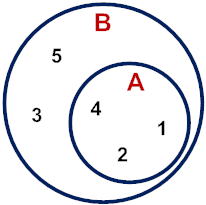Example 1: Given A = {1, 2, 4} and B = {1, 2, 3, 4, 5}, what is the relationship between these sets?

We say that A is a subset of B, since every element of A is also in B. This is denoted by: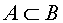Venn diagram for the relationship between these sets is shown to the right.

Answer: A is a subset of B.

Another way to define a subset is: A is a subset of B if every element of A is contained in BBoth definitions are demonstrated in the Venn diagram above.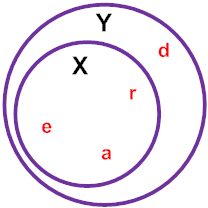Example 2: Given X = {a, r, e} and Y = {r, e, a, d}, what is the relationship between these sets?

We say that X is a subset of Y, since every element of X is also in Y. This is denoted by: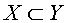A Venn diagram for the relationship between these sets is shown to the right.

Answer: X is a subset of Y.

Example 3: Given P = {1, 3, 4} and Q = {2, 3, 4, 5, 6}, what is the relationship between these sets?

We say that P is not a subset of Q since not every element of P is not contained in Q. For example, we can see that 1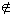Q. The statement "P is not a subset of Q" is denoted by: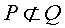Note that these sets do have some elements in common. The intersection of these sets is shown in the Venn diagram below.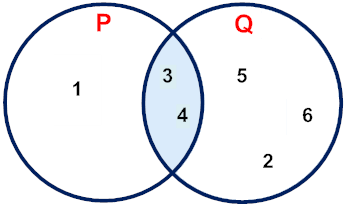Answer: P is not a subset of Q.

The notation for subsets is shown below.

 Symbol Meaningis a subset of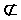is not a subset of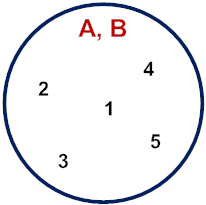Example 4: Given A = {1, 2, 3, 4, 5} and B = {3, 1, 2, 5, 4}, what is the relationship between A and B?

Analysis: Recall that the order in which the elements appear in a set is not important. Looking at the elements of these sets, it is clear that: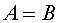Answer: A and B are equivalent.

Definition: For any two sets, if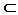B  andA, then A = B. Thus A and B are equivalent.

Example 5: List all subsets of the set C = {1, 2, 3}.

 Subset List all possible combinations of elements... D = {1} one at a time E = {2} one at a time F = {3} one at a time G = {1, 2} two at a time M = {1, 3} two at a time N = {2, 3} two at a time P = {1, 2, 3} three at a time Ø The null set has no elements.

Looking at example 5, you may be wondering why the null set is listed as a subset of C. There are no elements in a null set, so there can be no elements in the null set that aren't contained in the complete set. Therefore, the null set is a subset of every set. You may also be wondering: Is a set a subset of itself? The answer is yes: Any set contains itself as a subset. This is denoted by:A.

A subset that is smaller than the complete set is referred to as a proper subset. So the set {1, 2} is a proper subset of the set  {1, 2, 3} because the element 3 is not in the first set. In example 5, you can see that G is a proper subset of C, In fact, every subset listed in example 5 is a proper subset of C, except P. This is because and C are equivalent sets (P = C). Some mathematicians use the symbolto denote a subset and the symbolto denote a proper subset, with the definition for proper subsets as follows:

If AB, and A  B, then A is said to be a proper subset of B and it is denoted by AB.

While it is important to point out the information above, it can get a bit confusing, So let's think of subsets and proper subsets this way:

 Subsets and Proper Subsets The set {1, 2} is a proper subset of the set {1, 2, 3}. The set {1, 2, 3} is a not a proper subset of the set  {1, 2, 3}.

Do you see a pattern in the examples below?

Example 6: List all subsets of the set R = {x, y, z}. How many are there?

 Subsets D = {x} E = {y} F = {z} G = {x, y} H = {x, z} J = {y, z} K = {x, y, z} Ø

Answer: There are eight subsets of the set R = {x, y, z}.

Example 7: List all subsets of the set C = {1, 2, 3, 4}. How many are there?

 Subsets D = {1} M = {2, 4} E = {2} N = {3, 4} F = {3} O = {1, 2, 3} G = {4} P = {1, 2, 4} H = {1, 2} Q = {1, 3, 4} J = {1, 3} R = {2, 3, 4} K = {1, 4} S = {1, 2, 3, 4} L = {2, 3} Ø

Answer: There are 16 subsets of the set C = {1, 2, 3, 4}.

In example 6, set R has three (3) elements and eight (8) subsets. In example 7, set C has four (4) elements and 16 subsets. To find the number of subsets of a set with n elements, raise 2 to the nth power: That is:

The number of subsets in set A is 2, where n is the number of elements in set A.

L e s s o n   S u m m a r y

Subset: A is a subset of B: if every element of A is contained in B. This is denoted byB.

Equivalent Sets: For any two sets, ifB  andA, then A = B.

Null set: The null set is a subset of every set.

Sets and subsets: Any set contains itself as a subset. This is denoted byA.

Proper Subsets: If AB, and A  B, then A is said to be a proper subset of B and it is denoted by AB.

Number of Subsets: The number of subsets in set A is 2, where n is the number of elements in set A.

### Exercises

 2. Which of the following statements is true? {vowels}{consonants} {consonants}{vowels} {vowels}{alphabet} None of the above. RESULTS BOX: